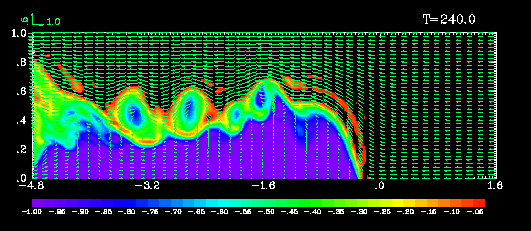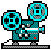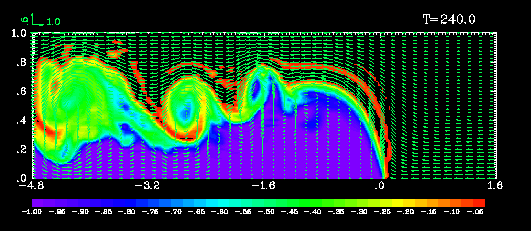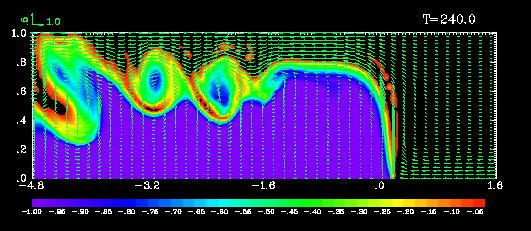### A Theoretical and Numerical Study of Density Currents in Non-constant Shear Flows

*Ming Xue, **Qin Xu and Ý*Kelvin K. Droegemeier

*Center for Analysis and Prediction of Storms
**Cooperative Institute for Mesoscale Meteorological Studies
ÝSchool of Meteorology
University of Oklahoma, Norman, Oklahoma 73019

(Submitted to Journal of the Atmospheric Sciences, July, 1996)

### ABSTRACT

The previous idealized two-fluid model of density current in constant shear is extended to the case where the inflow shear is confined to the low levels. The analytical solution is determined by the conservation of mass, momentum, vorticity and energy. It is found that a low-level shear acts in a similar manner to a uniform vertical shear in controlling the depth of a steady-state density current. When the shear enhances the low-level flow against the density current propagation, the current is deeper than half of the domain depth. Time-dependent numerical experiments are conducted for a variety of parameter settings, including various depths and strengths of the shear layer. The numerical results agree closely with the theoretical analyses.

Numerical experiments are also performed for a case where the initial depth of the density current is set to be comparable to the low-level shear, which is much shallower than that given by the steady-state solution. The circulation at the density current head remains shallow and is non-steady in this case, whereas the time averaged flow still exhibits a deep jump updraft pattern that is close to the theoretical solution, suggesting the applicability of the theoretical results to even more transient flows.

The simulated flow features are discussed in terms of balanced and unbalanced dynamics, and in the context of forcing and uplifting at the frontal zone in long-lived convective systems. Here the term balance refers to a flow configuration that satisfies the steady-state solution of the idealized theoretical model.

Underload the full length paper in PDF format.Figure 1. Schema of the steady-state model of a density current circulation in an environmental flow with low-level shear.### Experiment LS1 (Moderate positive low-level inflow shear. Shear strength alpha = 1.0, shear depth = 0.2, predicted density current depth = 0.59, simulated current depth=0.54). Shown in the figure are buoyancy contours and wind vectors at non-dimensional time 24.For a GIF movie, click on the image.### Experiment LS2 (Moderate negative low-level inflow shear. Shear strength alpha = -1.0, shear depth = 0.2, predicted density current depth = 0.41, simulated current depth=0.33). Shown in the figure are buoyancy contours and wind vectors at non-dimensional time 24.For a GIF movie, click on the image.### Experiment LS1A (Moderate positive low-level inflow shear. Shear strength alpha = 1.0, shear depth = 0.2, predicted density current depth = 0.683, simulated current depth=0.638).For a GIF movie, click on the image.### Experiment DS (Deep positive inflow shear. Shear strength alpha = 1.0, shear depth = 0.5, predicted density current depth = 0.68, simulated current depth=0.64).For a GIF movie, click on the image.### Experiment SLS (Strong positive low-level inflow shear. Shear strength alpha = 3.0, shear depth = 0.2, predicted density current depth = 0.77, simulated current depth=0.76).For a GIF movie, click on the image.